# Chapter 21 Gausss Law Electric Field Lines Electric

• Slides: 40Chapter 21 Gauss’s Law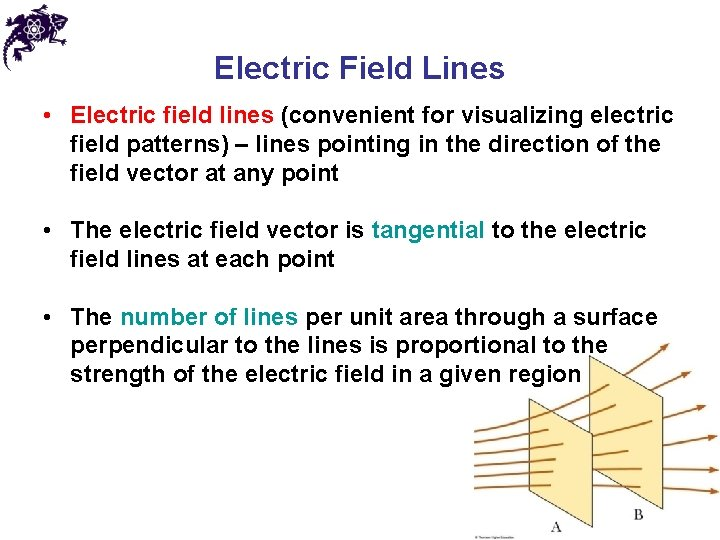Electric Field Lines • Electric field lines (convenient for visualizing electric field patterns) – lines pointing in the direction of the field vector at any point • The electric field vector is tangential to the electric field lines at each point • The number of lines per unit area through a surface perpendicular to the lines is proportional to the strength of the electric field in a given regionElectric Field Line Patterns • For a positive point charge the lines will radiate outward equally in all directions • For a negative point charge the lines will point inward equally in all directionsElectric Field Line Patterns • An electric dipole consists of two equal and opposite point charges • The number of field lines leaving the positive charge equals the number of lines terminating on the negative charge • For two equal but like point charges, at a great distance from the charges, the field would be approximately that of a single charge of 2 q (bulging out of the field lines between the charges – repulsion)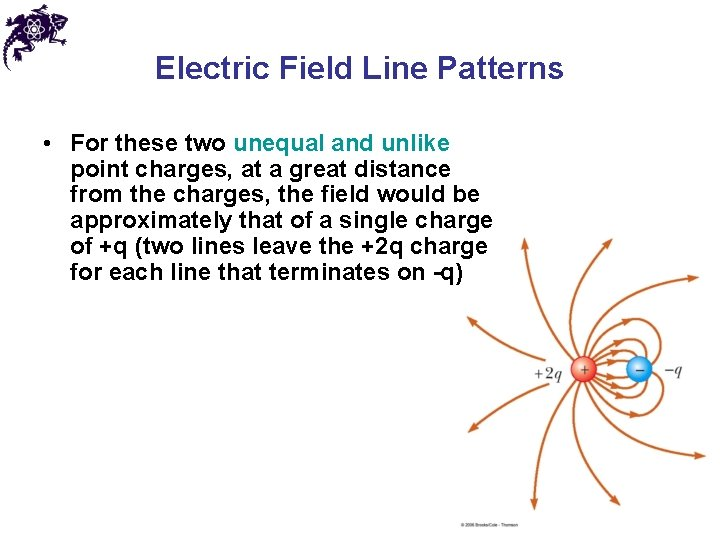Electric Field Line Patterns • For these two unequal and unlike point charges, at a great distance from the charges, the field would be approximately that of a single charge of +q (two lines leave the +2 q charge for each line that terminates on -q)Rules for Drawing Electric Field Lines • For a group of charges, the lines must begin on positive charges and end on negative charges • In the case of an excess of charge, some lines will begin or end infinitely far away • The number of lines drawn leaving a positive charge or ending on a negative charge is proportional to the magnitude of the charge • No two field lines can cross each otherElectric Flux • Electric flux is the product of the magnitude of the electric field and the surface area, A, perpendicular to the field ΦE = EA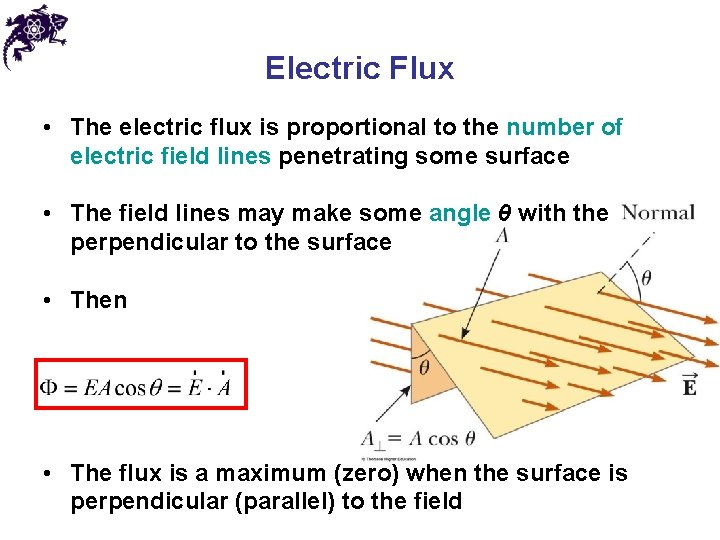Electric Flux • The electric flux is proportional to the number of electric field lines penetrating some surface • The field lines may make some angle θ with the perpendicular to the surface • Then • The flux is a maximum (zero) when the surface is perpendicular (parallel) to the fieldElectric Flux • If the field varies over the surface, Φ = EA cos θ is valid for only a small element of the area • In the more general case, look at a small area element • In general, this becomesElectric Flux • The surface integral means the integral must be evaluated over the surface in question • The value of the flux depends both on the field pattern and on the surface • SI units: N. m 2/C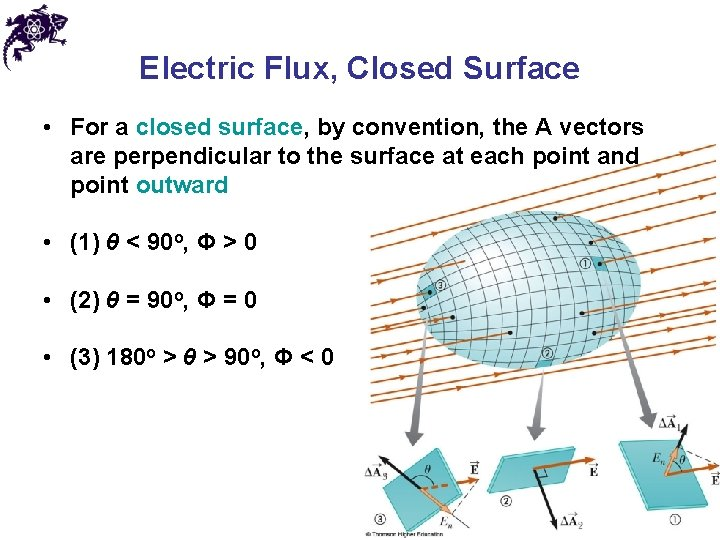Electric Flux, Closed Surface • For a closed surface, by convention, the A vectors are perpendicular to the surface at each point and point outward • (1) θ < 90 o, Φ > 0 • (2) θ = 90 o, Φ = 0 • (3) 180 o > θ > 90 o, Φ < 0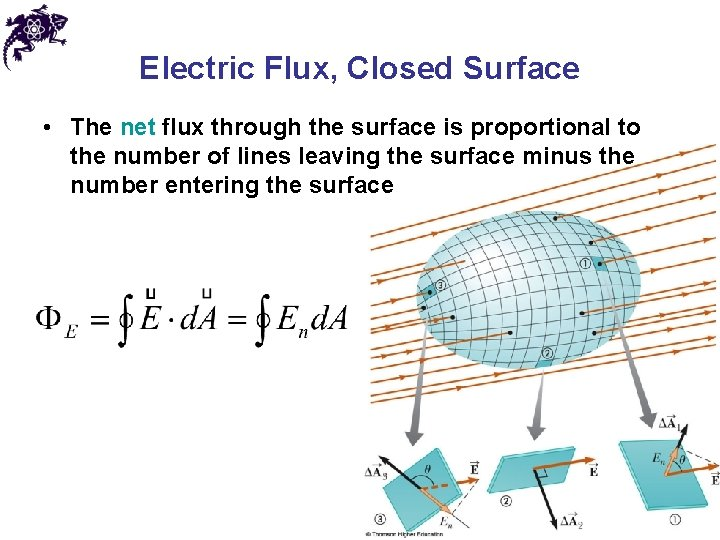Electric Flux, Closed Surface • The net flux through the surface is proportional to the number of lines leaving the surface minus the number entering the surface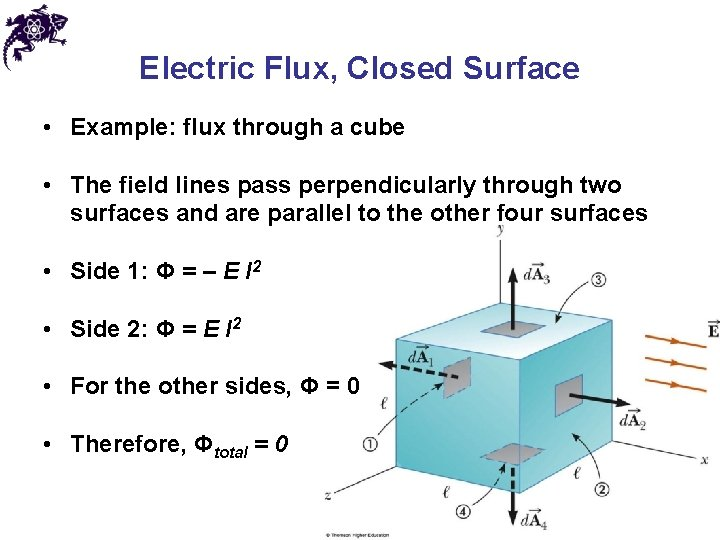Electric Flux, Closed Surface • Example: flux through a cube • The field lines pass perpendicularly through two surfaces and are parallel to the other four surfaces • Side 1: Φ = – E l 2 • Side 2: Φ = E l 2 • For the other sides, Φ = 0 • Therefore, Φtotal = 0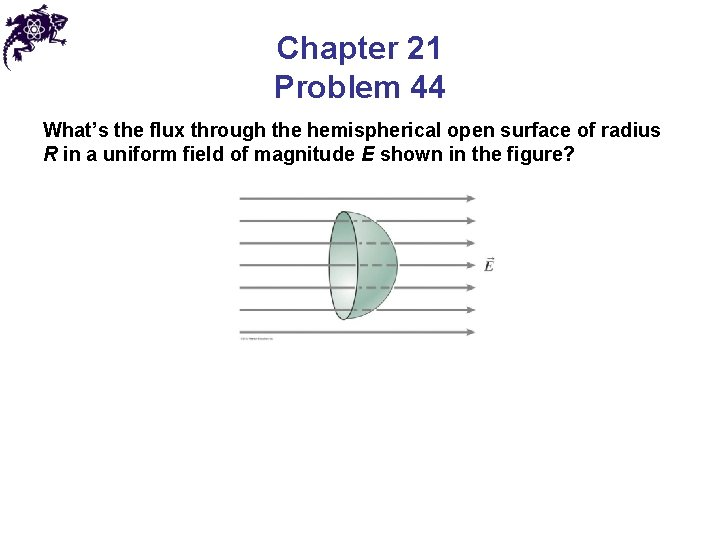Chapter 21 Problem 44 What’s the flux through the hemispherical open surface of radius R in a uniform field of magnitude E shown in the figure?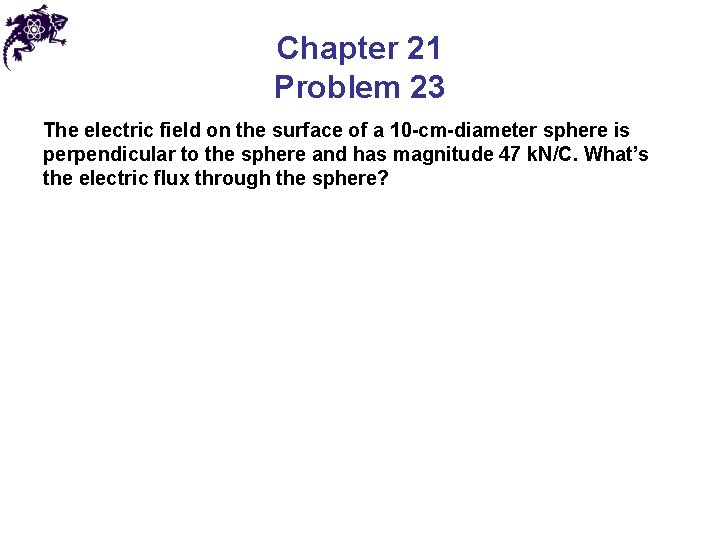Chapter 21 Problem 23 The electric field on the surface of a 10 -cm-diameter sphere is perpendicular to the sphere and has magnitude 47 k. N/C. What’s the electric flux through the sphere?Gauss’ Law Carl Friedrich Gauss 1777 – 1855 • Gauss’ Law: electric flux through any closed surface is proportional to the net charge Q inside the surface • εo = 8. 85 x 10 -12 C 2/Nm 2 : permittivity of free space • The area in Φ is an imaginary Gaussian surface (does not have to coincide with the surface of a physical object)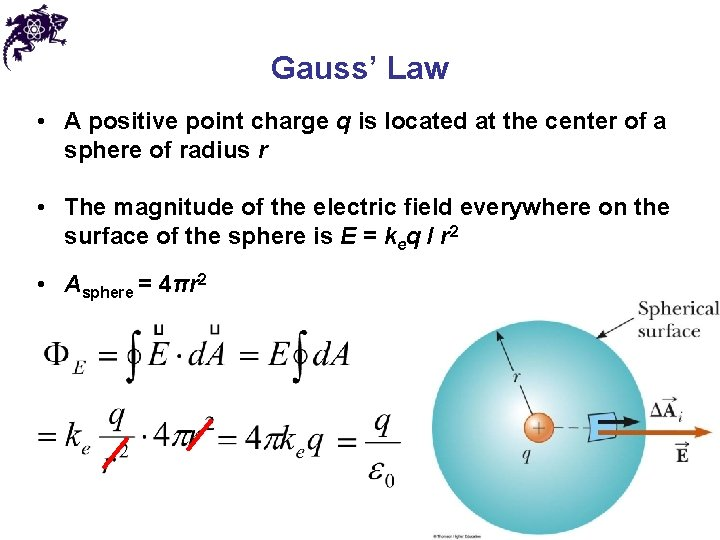Gauss’ Law • A positive point charge q is located at the center of a sphere of radius r • The magnitude of the electric field everywhere on the surface of the sphere is E = keq / r 2 • Asphere = 4πr 2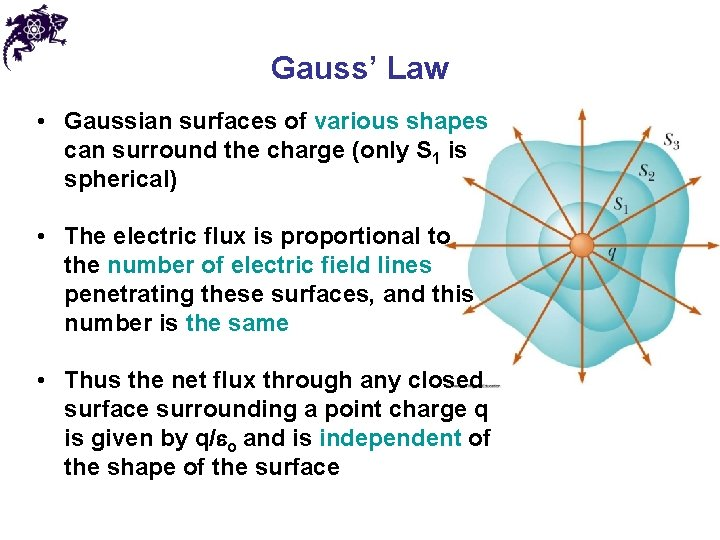Gauss’ Law • Gaussian surfaces of various shapes can surround the charge (only S 1 is spherical) • The electric flux is proportional to the number of electric field lines penetrating these surfaces, and this number is the same • Thus the net flux through any closed surface surrounding a point charge q is given by q/eo and is independent of the shape of the surfaceGauss’ Law • If the charge is outside the closed surface of an arbitrary shape, then any field line entering the surface leaves at another point • Thus the electric flux through a closed surface that surrounds no charge is zero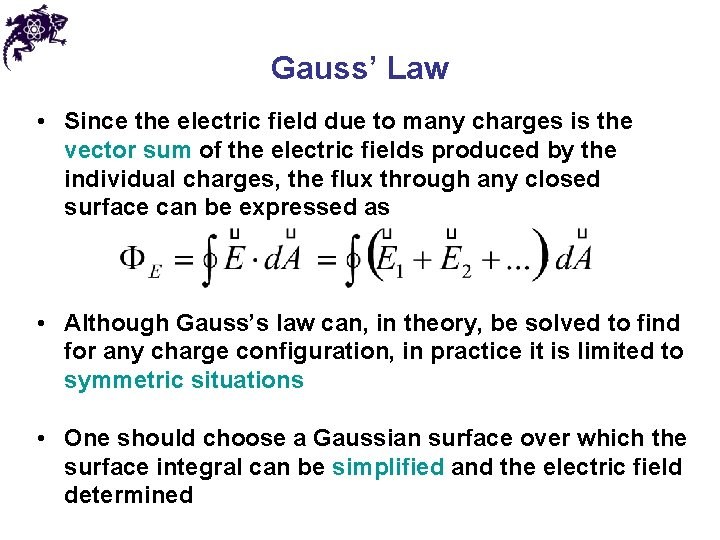Gauss’ Law • Since the electric field due to many charges is the vector sum of the electric fields produced by the individual charges, the flux through any closed surface can be expressed as • Although Gauss’s law can, in theory, be solved to find for any charge configuration, in practice it is limited to symmetric situations • One should choose a Gaussian surface over which the surface integral can be simplified and the electric field determined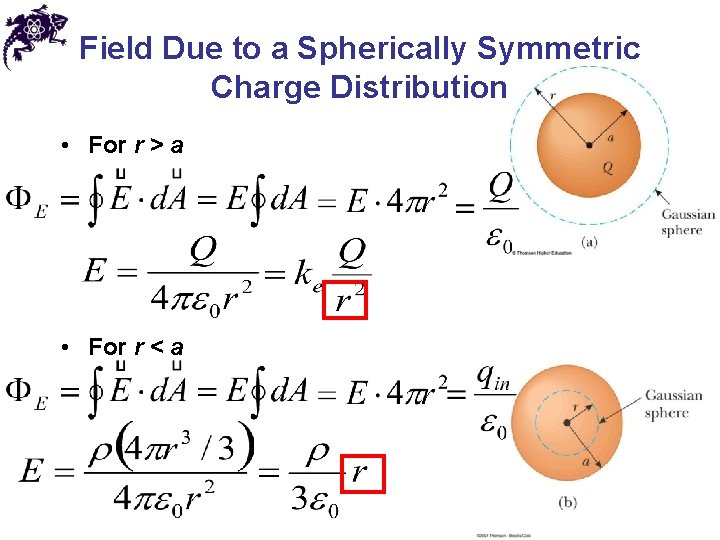Field Due to a Spherically Symmetric Charge Distribution • For r > a • For r < aField Due to a Spherically Symmetric Charge Distribution • Inside the sphere, E varies linearly with r (E → 0 as r → 0) • The field outside the sphere is equivalent to that of a point charge located at the center of the sphere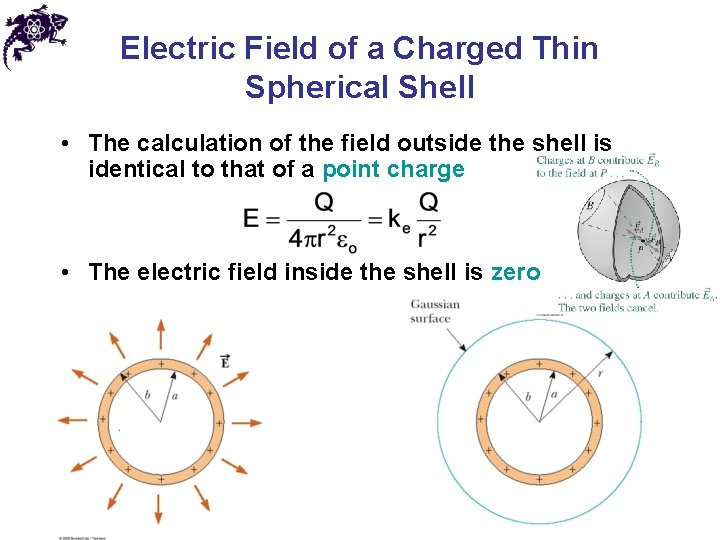Electric Field of a Charged Thin Spherical Shell • The calculation of the field outside the shell is identical to that of a point charge • The electric field inside the shell is zeroField Due to a Line of Charge • Select a cylindrical Gaussian surface (of radius r and length ℓ) • Electric field is constant in magnitude and perpendicular to the surface at every point on the curved part of the surface • The end view confirms the field is perpendicular to the curved surface • The field through the ends of the cylinder is 0 since the field is parallel to these surfaces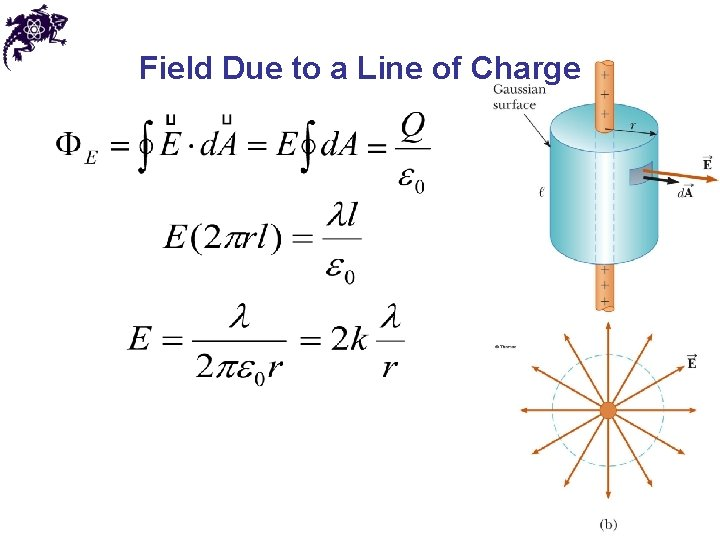Field Due to a Line of ChargeField Due to a Plane of Charge • The uniform field must be perpendicular to the sheet and directed either toward or away from the sheet • Use a cylindrical Gaussian surface • The flux through the ends is EA and there is no field through the curved part of the surface • Surface charge density σ = Q / A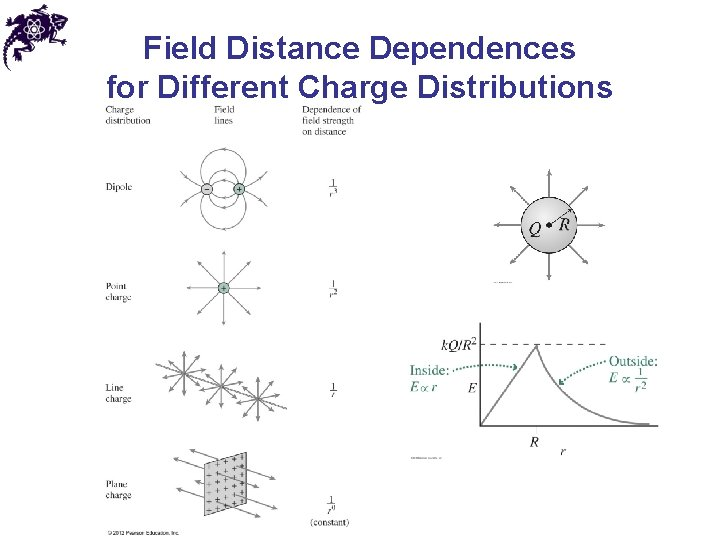Field Distance Dependences for Different Charge Distributions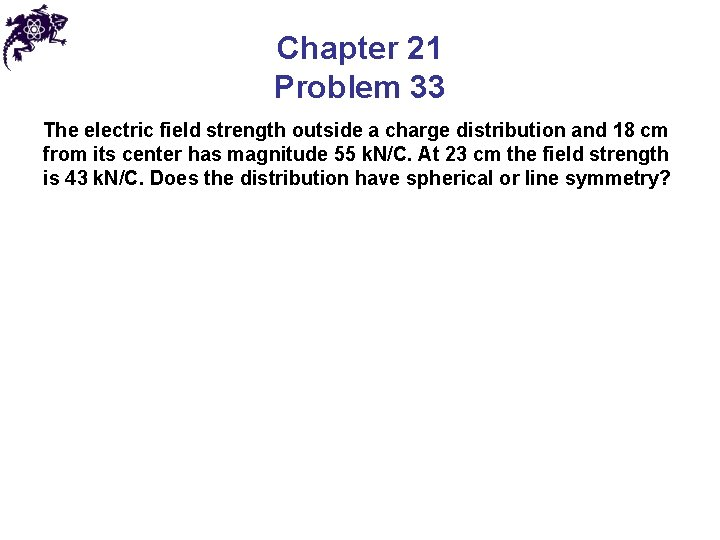Chapter 21 Problem 33 The electric field strength outside a charge distribution and 18 cm from its center has magnitude 55 k. N/C. At 23 cm the field strength is 43 k. N/C. Does the distribution have spherical or line symmetry?Conductors in Electrostatic Equilibrium • When no net motion of charge occurs within a conductor, the conductor is said to be in electrostatic equilibrium • An isolated conductor has the following properties: • Property 1: The electric field is zero everywhere inside the conducting material • If this were not true there were an electric field inside the conductor, the free charge there would move and there would be a flow of charge – the conductor would not be in equilibrium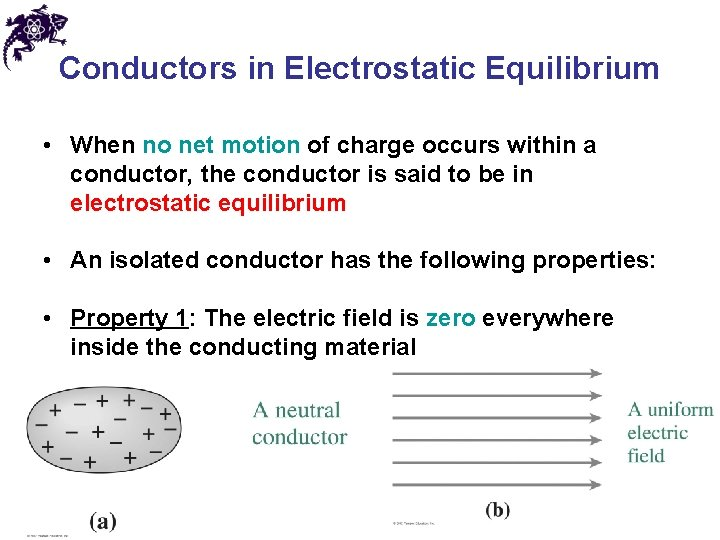Conductors in Electrostatic Equilibrium • When no net motion of charge occurs within a conductor, the conductor is said to be in electrostatic equilibrium • An isolated conductor has the following properties: • Property 1: The electric field is zero everywhere inside the conducting material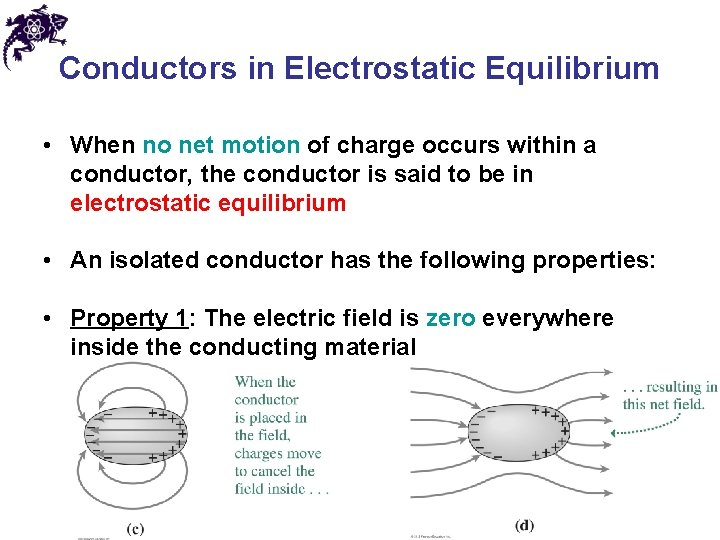Conductors in Electrostatic Equilibrium • When no net motion of charge occurs within a conductor, the conductor is said to be in electrostatic equilibrium • An isolated conductor has the following properties: • Property 1: The electric field is zero everywhere inside the conducting material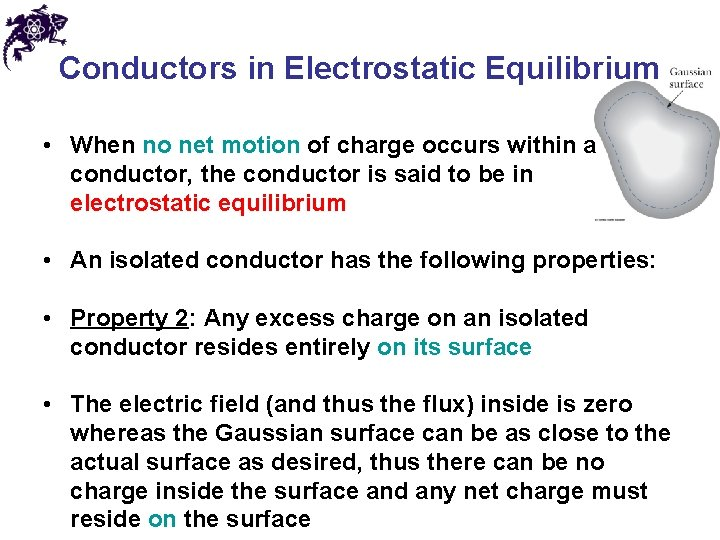Conductors in Electrostatic Equilibrium • When no net motion of charge occurs within a conductor, the conductor is said to be in electrostatic equilibrium • An isolated conductor has the following properties: • Property 2: Any excess charge on an isolated conductor resides entirely on its surface • The electric field (and thus the flux) inside is zero whereas the Gaussian surface can be as close to the actual surface as desired, thus there can be no charge inside the surface and any net charge must reside on the surface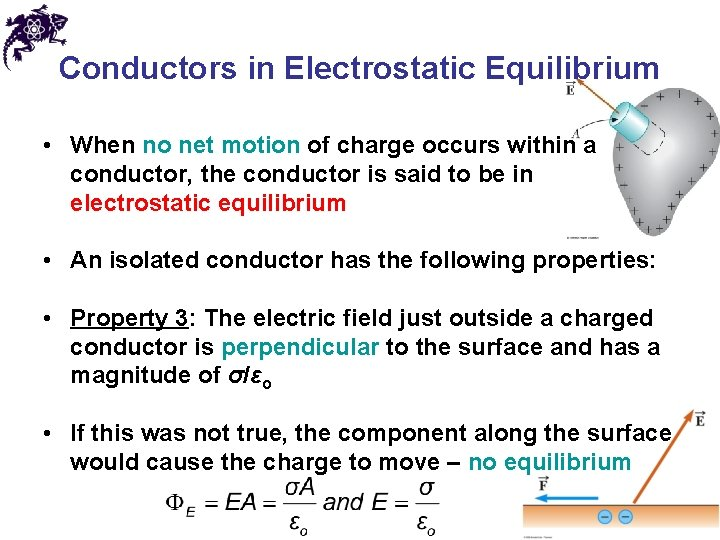Conductors in Electrostatic Equilibrium • When no net motion of charge occurs within a conductor, the conductor is said to be in electrostatic equilibrium • An isolated conductor has the following properties: • Property 3: The electric field just outside a charged conductor is perpendicular to the surface and has a magnitude of σ/εo • If this was not true, the component along the surface would cause the charge to move – no equilibriumConductors in Electrostatic Equilibrium • When no net motion of charge occurs within a conductor, the conductor is said to be in electrostatic equilibrium • An isolated conductor has the following properties: • Property 4: On an irregularly shaped conductor, the charge accumulates at locations where the radius of curvature of the surface is smallest • The forces from the charges at the sharp end produce a larger resultant force away from the surface.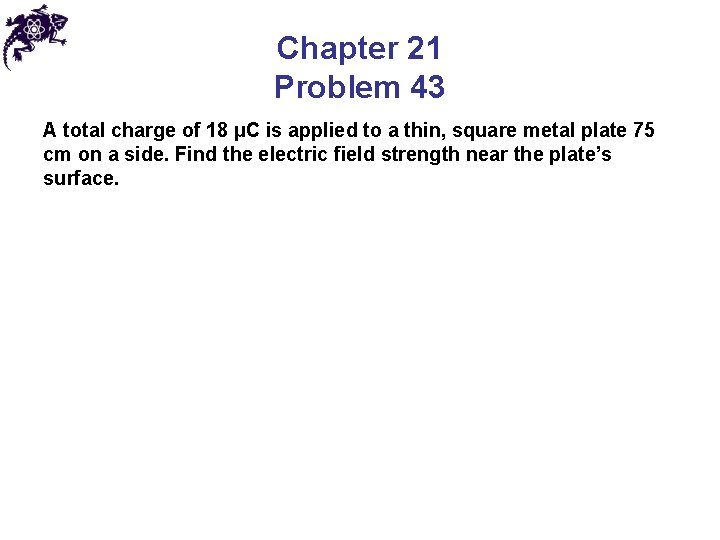Chapter 21 Problem 43 A total charge of 18 µC is applied to a thin, square metal plate 75 cm on a side. Find the electric field strength near the plate’s surface.Answers to Even Numbered Problems Chapter 21: Problem 22 ± 490 N ⋅ m 2/C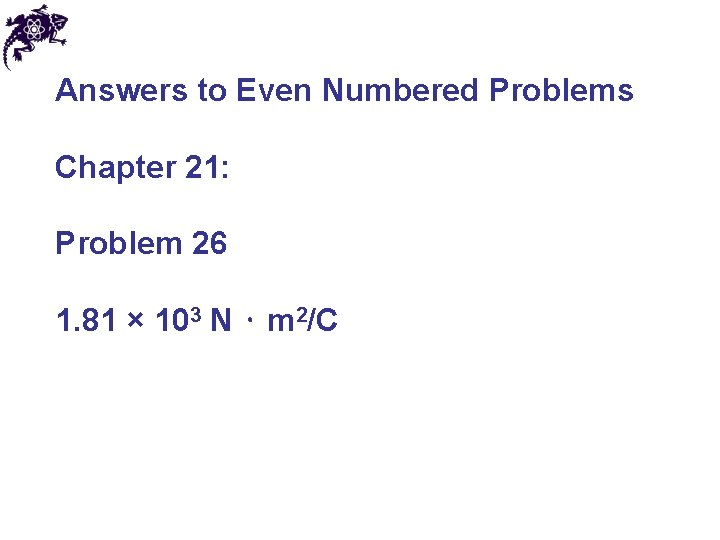Answers to Even Numbered Problems Chapter 21: Problem 26 1. 81 × 103 N ⋅ m 2/CAnswers to Even Numbered Problems Chapter 21: Problem 34 − 2. 0 × 10− 4 C/m 2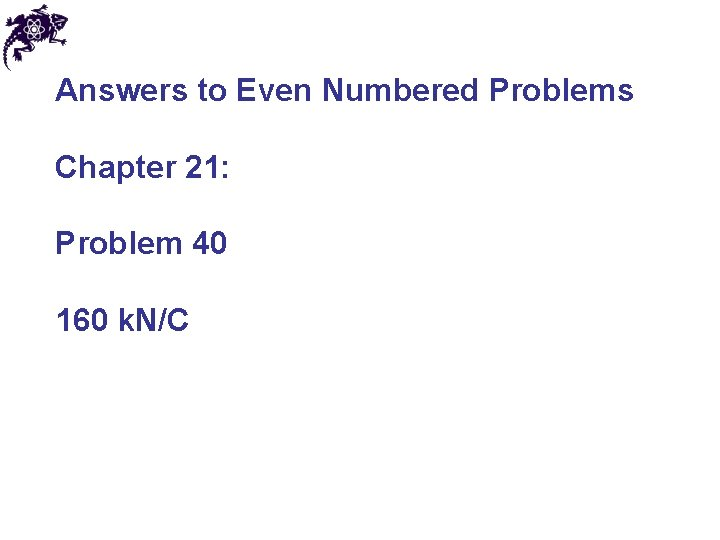Answers to Even Numbered Problems Chapter 21: Problem 40 160 k. N/CAnswers to Even Numbered Problems Chapter 21: Problem 58 58 n. C/m 2